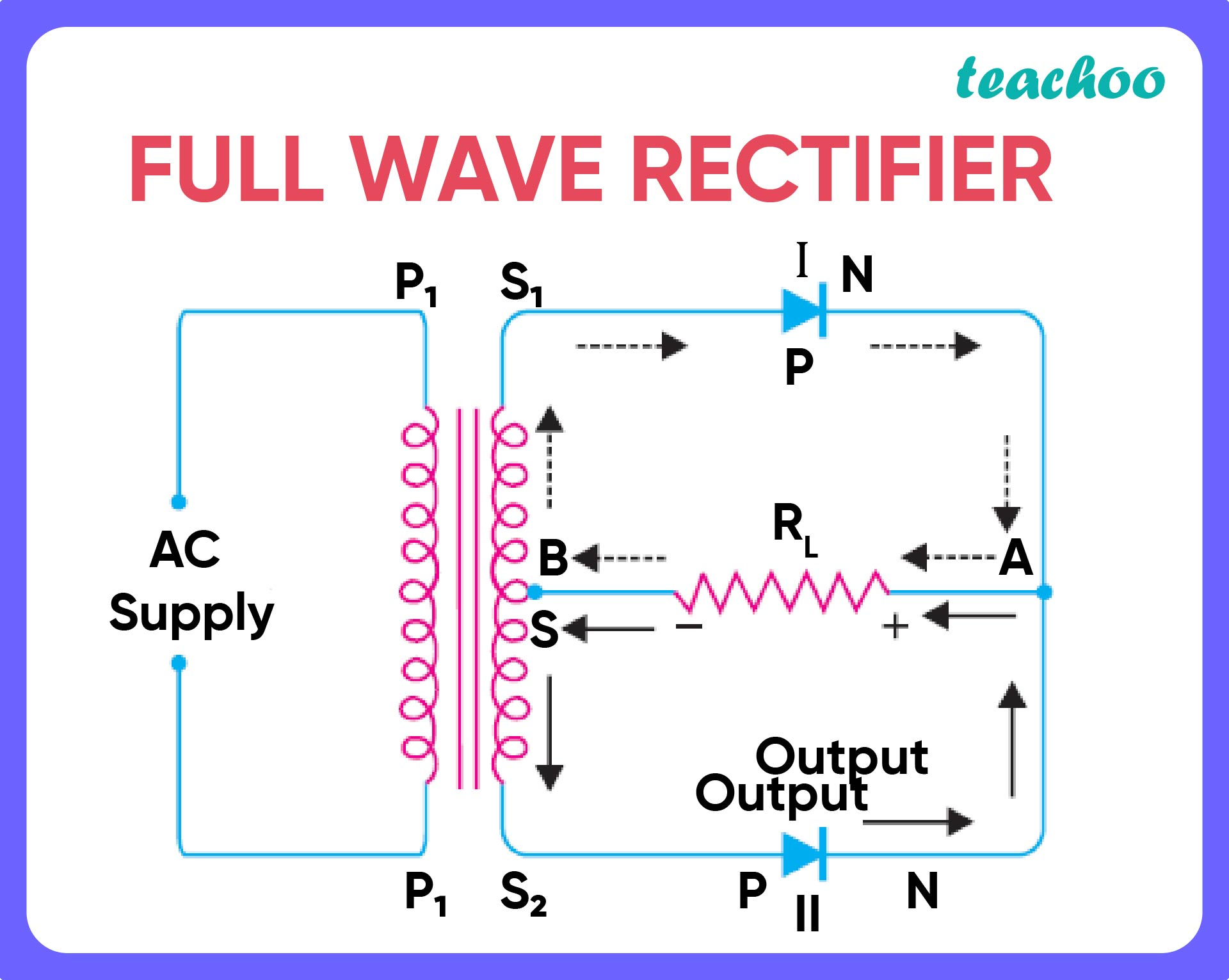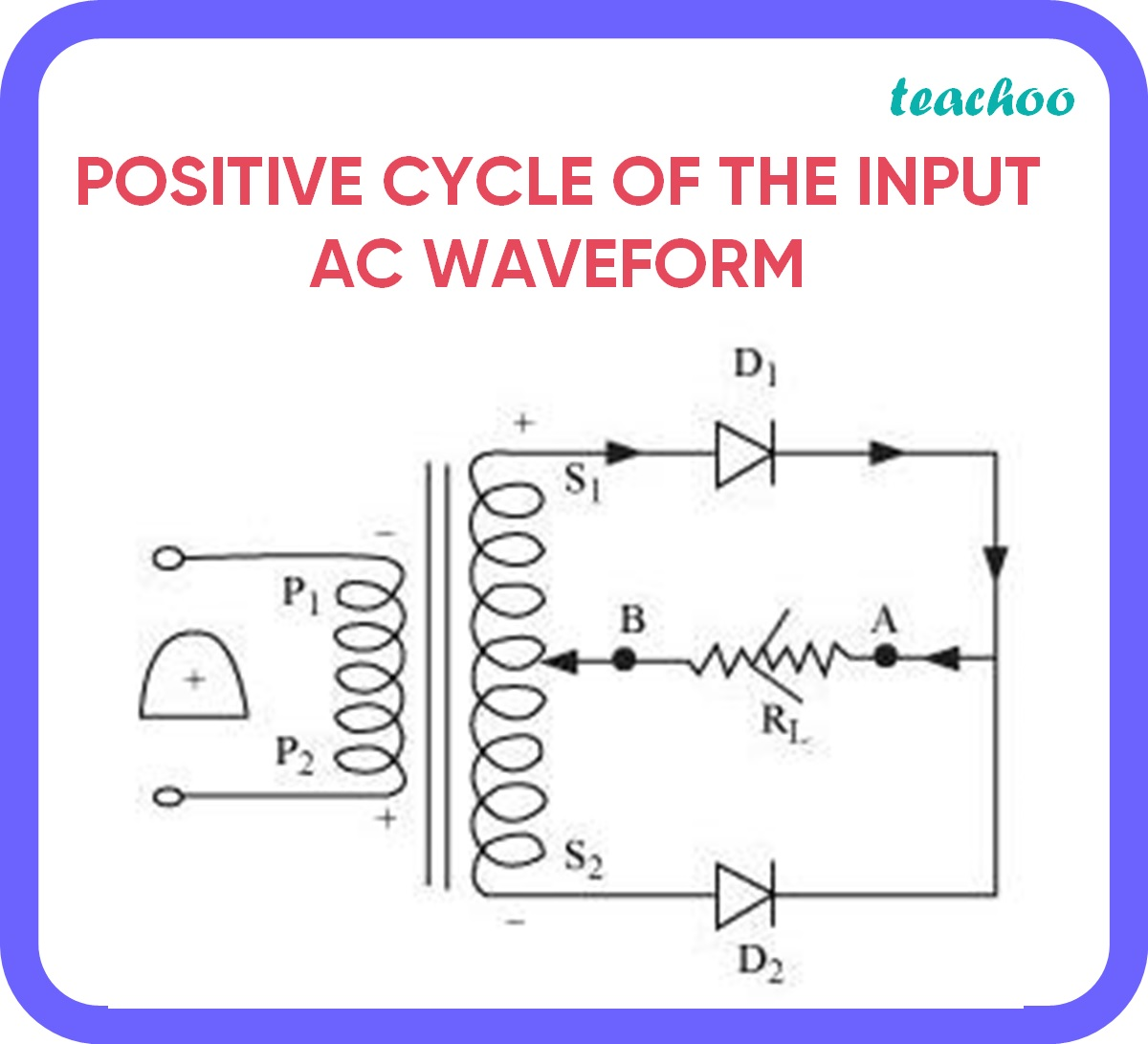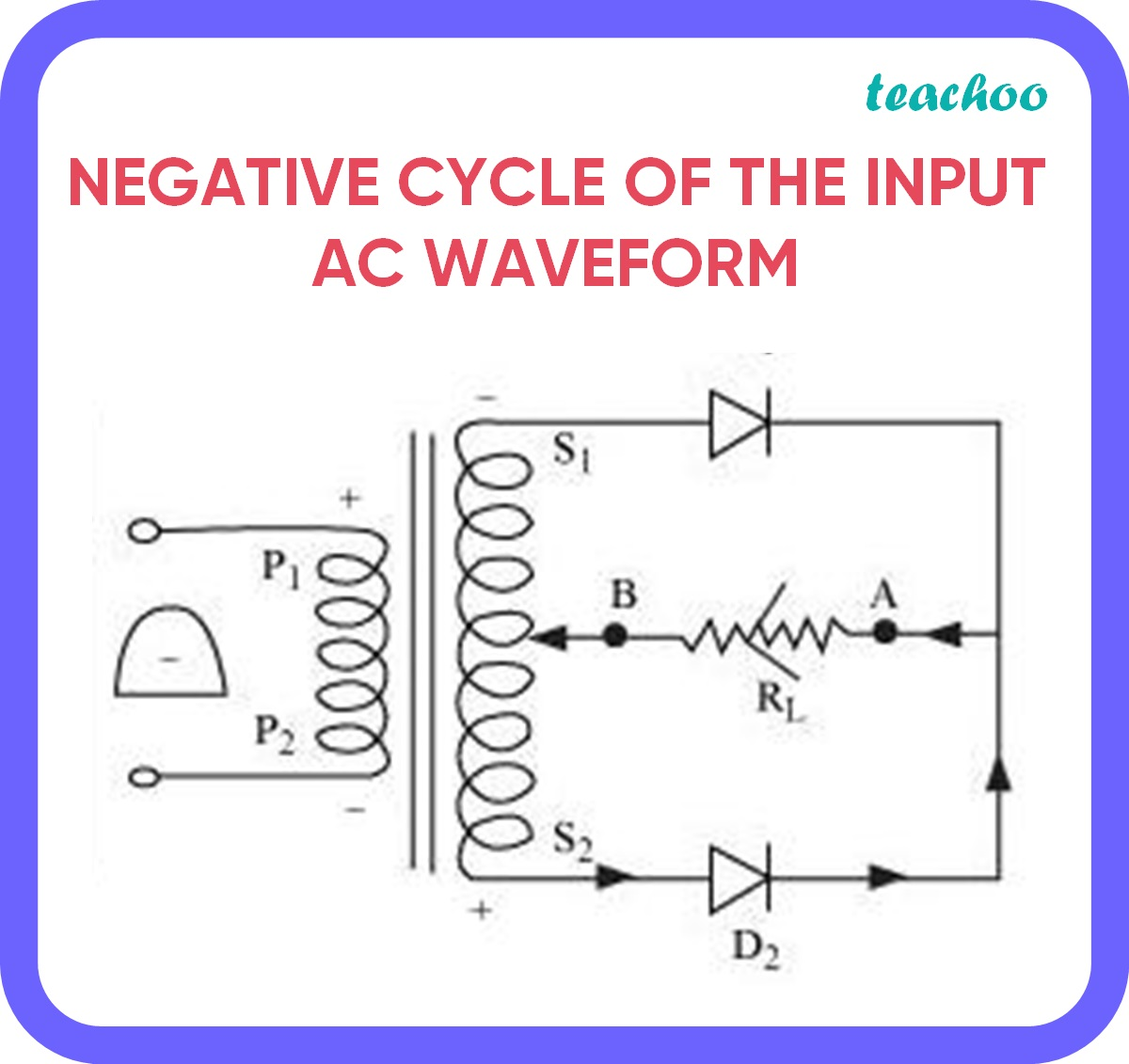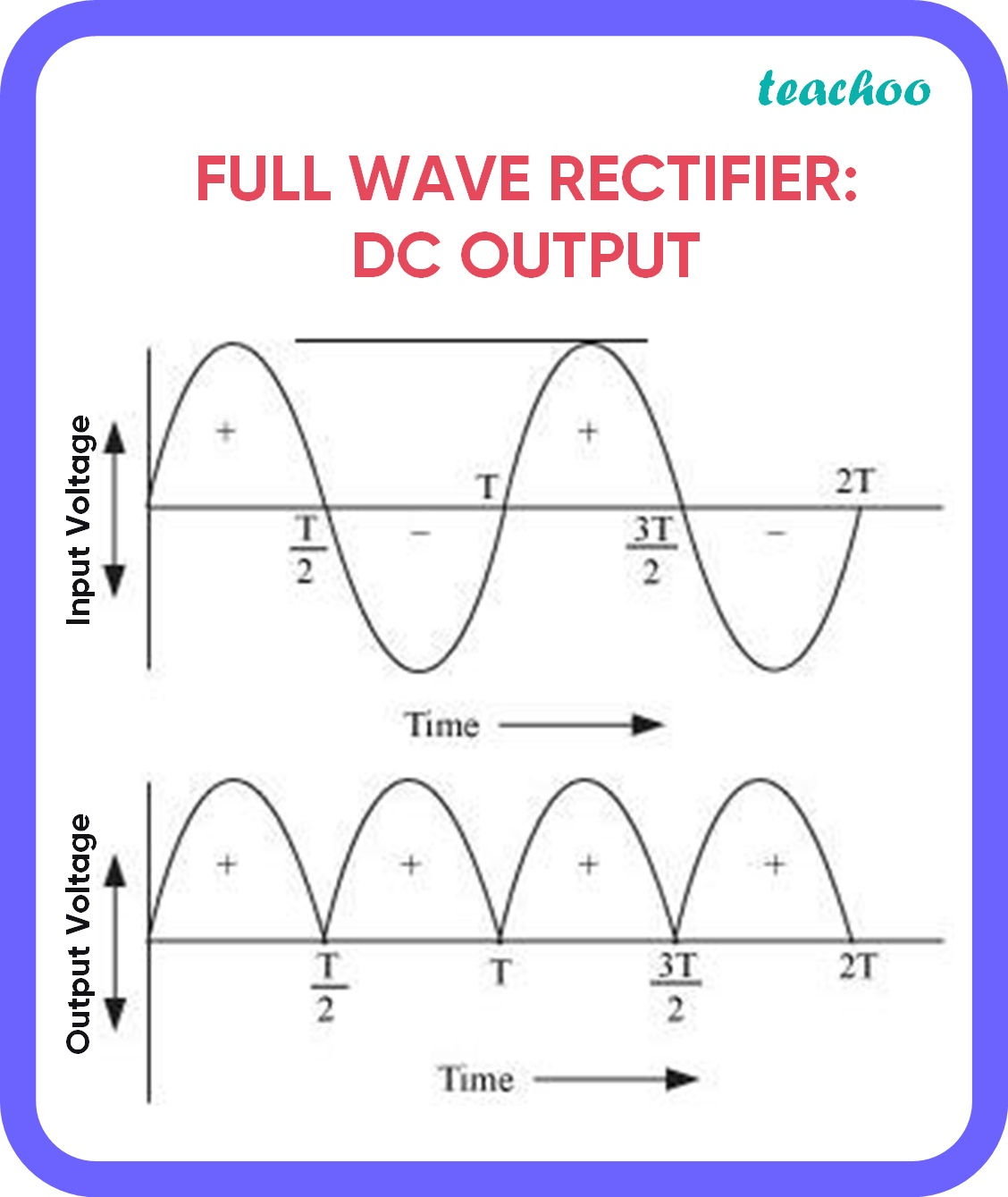CBSE Class 12 Sample Paper for 2022 Boards [Term 2] - Physics

Physics Class 12
Solutions to CBSE Sample Paper - Physics Class 12

## Explain with a proper diagram how an ac signal can be converted into dc ( pulsating)signal with output frequency as double than the input frequency using pn junction diode. Give its input and output waveforms.

A p-n junction diode is formed by combining a p-type and a n-type semiconductor.

• A p-n junction diode allows current to pass only when it is forward biased
• This property is used to rectify alternating voltages and the circuit used for this purpose is called a rectifier .
• AC current becomes DC when it stops changing directions in a set period of time. That is, DC current has a constant direction .
• A rectifier that produces an output signal with frequency as double than the input frequency is known as a full wave rectifier

The diagram is as given below:• The input is given across the primary coil .
• The secondary coil of the transformer receives the signal
• Output is taken across resistor R L

(a) In the positive cycle of the input AC waveform:• Diode D1 is forward biased - it acts as a short circuit
• Diode D2 is reversed biased - it acts as an open circuit

Hence, current flows through R L and is observed as output.

(b) In the negative cycle of the input AC waveform:• Diode D1 is reversed biased - it acts as a open circuit
• Diode D2 is forward biased - it acts as an short circuit

Hence, current flows through  R L and is observed as output.

You may notice that the direction of current in the first case is clockwise, while the second case is anti-clockwise.

• The current produced is DC because the direction of the current in the load resistor in both cases is the same
• Since, the no. of cycles per second has doubled , a full wave rectifier produces an output with double the input frequency.Learn in your speed, with individual attention - Teachoo Maths 1-on-1 Class